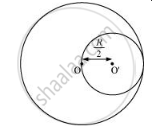CBSE (Science) Class 11CBSE
Share

# From a Uniform Disk of Radius R, a Circular Hole of Radius R/2 is Cut Out. the Centre of the Hole is at R/2 from the Centre of the Original Disc. Locate the Centre of Gravity of the Resulting Flat Body. - CBSE (Science) Class 11 - Physics

ConceptEquilibrium of Rigid Bodies

#### Question

From a uniform disk of radius R, a circular hole of radius R/2 is cut out. The centre of the hole is at R/2 from the centre of the original disc. Locate the centre of gravity of the resulting flat body.

#### Solution 1

R/6; from the original centre of the body and opposite to the centre of the cut portion.

Mass per unit area of the original disc = σ

Radius of the original disc = R

Mass of the original disc, M = πR2σ

The disc with the cut portion is shown in the following figure:Radius of the smaller disc = R/2

Mass of the smaller disc, M  = pi(R/2)^2 sigma = 1/4piR^2 sigma = M/4

Let O and O′ be the respective centres of the original disc and the disc cut off from the original. As per the definition of the centre of mass, the centre of mass of the original disc is supposed to be concentrated at O, while that of the smaller disc is supposed to be concentrated at O′.

It is given that:

OO' = R/2

After the smaller disc has been cut from the original, the remaining portion is considered to be a system of two masses. The two masses are:

M (concentrated at O), and -M(=M/4)Concentrated at O'

(The negative sign indicates that this portion has been removed from the original disc.)

Let x be the distance through which the centre of mass of the remaining portion shifts from point O.

The relation between the centres of masses of two masses is given as:

x = (m_1r_1 + m_2r_2)/(m_1+m_2)

For the given system, we can write:

x = (Mxx0-Mxx(R/2))/(M+(-M'))

=(-M/4 xxR/2)/(M - M/4) = (-MR)/8 xx 4/(3M) = -R/6

(The negative sign indicates that the centre of mass gets shifted toward the left of point O.)

#### Solution 2

Let from a bigger uniform disc of radius R with centre O a smaller circular hole of radius R/2 with its centre at O1(where R OO1 = R/2) is cut out. Let centre of gravity or the centre of mass of remaining flat body be at O2, where OO2 = x. If σ be mass per unit area, then mass of whole disc M1 = πR2σ and mass of cut out part

M_2 = pi(R/2)^2 sigma = 1/4piR^2 sigma = M_1/4

x = (M_1xx(0) - M_2(OO_1))/(M_1-M_2) = (0 - M_1/4 xx R/2)/(M_1 - M_1/4) = -R/6

i.e., O2 is at a distance R/6 from centre of disc on diametrically opposite side to centre of hole

Is there an error in this question or solution?

#### APPEARS IN

NCERT Solution for Physics Textbook for Class 11 (2018 to Current)
Chapter 7: System of Particles and Rotational Motion
Q: 16 | Page no. 179
Solution From a Uniform Disk of Radius R, a Circular Hole of Radius R/2 is Cut Out. the Centre of the Hole is at R/2 from the Centre of the Original Disc. Locate the Centre of Gravity of the Resulting Flat Body. Concept: Equilibrium of Rigid Bodies.
S